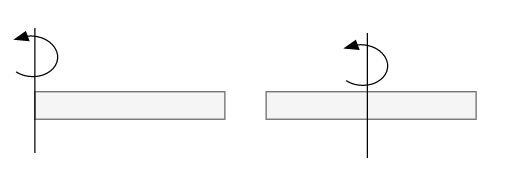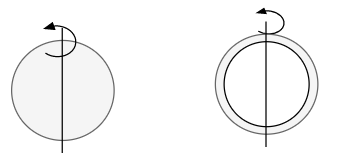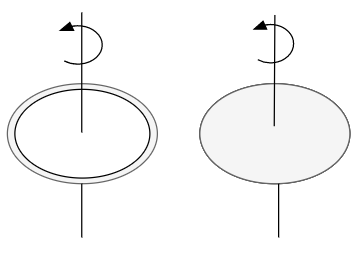# Moment of Inertia calculator

## What is Moment of Inertia

• Moment of inertia of a system about axis of rotation is given as
$I=\sum m_i r_i^2$
where $m_i$ is the mass of the ith particle and $r_i$ is its perpendicular distance from the axis of rotation
• For a system consisting of collection of discrete particles ,above equation can be used directly for calculating the moment of inertia
• For continuous bodies ,moment of inertia about a given line can be obtained using integration technique
• SI unit of Moment of inertia is $Kg m^2$
• It is a scalar quantity
Some Important formulas
1. Moment of Inertia calculator for a mass m at distance d from axis of Rotation is given by
$I = md^2$
Where
m -> mass
d -> distance d from axis of Rotation
I -> Moment of inertia
2. Moment of Inertia for a thin rectangular rod around perpendicular bisector and perpendicular axis through one end is given by
$I_p = \frac {mL^2}{12}$
$I_e = \frac {mL^2}{3}$
Where
m -> Mass of the thin rectangular rod
L -> Length of the Rod
$I_p$ -> Moment of Inertia around perpendicular bisector
$I_e$ -> Moment of Inertia around perpendicular axis through one end
3. Moment of Inertia for a solid and hollow sphere about the axis through its center is given by
$I_S = \frac {2}{5}mR^2$
$I_H = \frac {2}{3}mR^2$
Where
m -> Mass of the Sphere
R -> Radius of the sphere
$I_S$ -> Moment of Inertia of Solid sphere about the axis through its center
$I_H$ -> Moment of Inertia of Hollow sphere about the axis through its center
4.Moment of Inertia calculator for a thin or solid cylinder/disk is given
Moment of Inertia for Solid Disk or cylider about the central axis
$I_S = \frac {1}{2}mR^2$
Moment of Inertia for thin Disk or cylider about the central axis
$I_H = mR^2$

## Moment of Inertia calculator for a mass m at distance d from axis of Rotation

Note
• Enter the values of mass and distance
• Click on the calculate button.
The formula used for solving the question is
$I = md^2$

### Moment of Inertia calculator

Example of Few questions where you can use this Moment of Inertia Solver
Question 1
A particle of mass 5 kg is placed at a distance 5 m from the axis of rotation. Find the moment of inertia of particle about the axis of rotation
Solution
m = 5 kg, d=5 m, I=?
From the formula
$I = md^2$
$I=5 \times 5^2 = 125 \ kg m^2$

Question 2
A object of mass 2 kg is situated at a distance 10 m from the axis of rotation. Find the moment of inertia of about about the axis of rotation
Solution
m = 2 kg, d=10 m, I=?
From the formula
$I = md^2$
$I=2 \times 10^2 = 200 \ kg m^2$

## Moment of Inertia for a thin rectangular rod around perpendicular bisector and perpendicular axis through one endNote
• Enter the values of mass and length of the rod
• Click on the calculate button.
The formula used for solving the question is
$I_p = \frac {mL^2}{12}$
$I_e = \frac {mL^2}{3}$

### Moment of Inertia calculator

Example of Few questions where you can use this Moment of Inertia of thin rectangular rod calculator
Question 1
Calculate the Moment of inertia of thin rectangular rod of Mass 2 kg and Length 2 m around the perpendicular bisector and perpendicular axis through one end
Solution
m = 2 kg, L=2 m, $I_e$=?,$I_p$=?
Moment of inertia around perpendicular bisector is given by $I_p = \frac {mL^2}{12}$
$I_p = \frac {2 \times 2^2}{12}= 2/3 \ Kg m^2$br> Moment of inertia about perpendicular axis through one end is given by $I_e = \frac {mL^2}{3}$
$I_e = \frac {2 \times 2^2}{3} = 8/3 \ Kg m^2$br>
Question 2
A thin rectangular rod has Mass 1 kg and Length 1 m . Find the moment of inertia around the perpendicular bisector and perpendicular axis through one end
Solution
m = 1 kg, L=1 m, $I_e$=?,$I_p$=?
Moment of inertia around perpendicular bisector is given by $I_p = \frac {mL^2}{12}$
$I_p = \frac {1 \times 1^2}{12}= 1/12 \ Kg m^2$br> Moment of inertia about perpendicular axis through one end is given by $I_e = \frac {mL^2}{3}$
$I_e = \frac {1 \times 1^2}{3} = 1/3 \ Kg m^2$br>

## Moment of Inertia for a solid and hollow sphereNote
• Enter the values of mass and radius of the sphere
• Click on the calculate button.
The formula used for solving the question is
$I_S = \frac {2}{5}mR^2$
$I_H = \frac {2}{3}mR^2$

### Moment of Inertia calculator

Example of Few questions where you can use this Moment of Inertia of Sphere calculator
Question 1
Calculate the Moment of inertia of hollow sphere of Mass 2 kg and Diameter 6 m about the axis through its center
Solution
m = 2 kg, D=6 m, $I$=? Therefore R=3 m Moment of inertia about the axis through its center is given by $I_H = \frac {2}{3}mR^2$
$I_H = \frac {2}{3} \times 2 \times 3^2= 12 \ kg-m^2$

Question 2
A Solid Sphere has Mass 1 kg and Radius 5 m . Find the moment of inertia about the axis through its center
Solution
m = 1 kg,R=5 m, I=?
Moment of inertia about the axis through its center is given by $I_S = \frac {2}{5}mR^2$
$I_S = \frac {2}{5} \times 1 \times 5^2= 10 \ kg-m^2$

## Moment of Inertia calculator for a thin or solid cylinder/diskNote
• Enter the values of mass and radius of the sphere
• Click on the calculate button.
The formula used for solving the question is
Moment of Inertia for Solid Disk or cylinder about the central axis
$I_S = \frac {1}{2}mR^2$
Moment of Inertia for thin Disk or cylinder about the central axis
$I_H = mR^2$

### Moment of Inertia calculator

Example of Few questions where you can use this Moment of Inertia of thin/solid cylinder calculator
Question 1
Calculate the Moment of inertia of thin cylinder of Mass 10 kg , Diameter .6 m and Length =1 m about the central axis
Solution
m = 10 kg, D=.6 m, L=1 m,$I$=? Therefore R=.3 m Moment of Inertia for thin Disk or cylinder about the central axis
$I_H = mR^2$
$I_H = 10 \times .3^2= .9 \ kg-m^2$

Question 2
A Solid disk has Mass 1 kg and Radius .2 m . Find the moment of inertia about the central axis
Solution
m = 1 kg,R=.2 m, I=?
Moment of Inertia for Solid Disk or cylinder about the central axis
$I_S = \frac {1}{2}mR^2$
$I_S = \frac {1}{2} \times 1 \times (.2)^2= .02 \ kg-m^2$